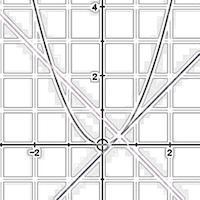# 5.2 & 5.4 Tangents, normals and the second derivativeIn this unit we look at find the equations of tangents and normals to curves, increasing and decreasing functions and the second derivative. All this builds on the fundamental notion that calculus tells us about the rate of of change of a function.

To access the contents of this site, you need to log in or subscribe